# Power Conservation in AC Circuits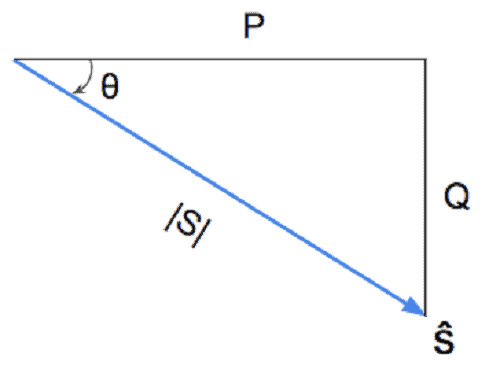This principle of conservation of power applies to ac circuits as well as to dc circuits.

Make sure to read what is ac circuit first.

## Conservation of AC Power

To see this, consider the circuit in Figure.(1a), where two load impedances Z1 and Z2 are connected in parallel across an ac source V.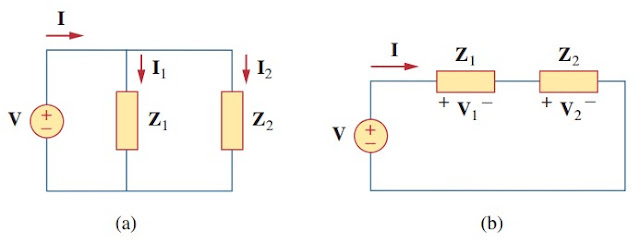Figure 1. An ac voltage source supplied loads connected in : (a) parallel, (b) series.

KCL gives(1)

The complex power supplied by the source is (from now on, unless otherwise specified, all values of voltages and currents will be assumed to be rms values)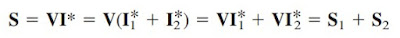(2)

where S1 and S2 denote the complex powers delivered to loads Z1 and Z2, respectively.

If the loads are connected in series with the voltage source, as shown in Figure.(1b), KVL yields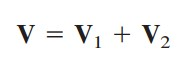(3)

The complex power supplied by the source is(4)

where S1 and S2 denote the complex powers delivered to loads Z1 and Z2, respectively.

We conclude from Equations.(2) and (4) that whether the loads are connected in series or in parallel (or in general), the total power supplied by the source equals the total power delivered to the load. Thus, in general, for a source connected to N loads.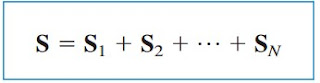(5)

This means that the total complex power in a network is the sum of the complex powers of the individual components. (This is also true of real power and reactive power, but not true of apparent power). This expresses the principle of conservation of ac power:

The complex, real, and reactive powers of the sources equal the respective sums of the complex, real, and reactive powers of the individual loads.

From this we imply that the real (or reactive) power flow from sources in a network equals the real (or reactive) power flow into the other elements in the network.

## Conservation of AC Power Examples

For better understanding let us review the examples below :
1. Figure.(2) shows a load being fed by a voltage source through a transmission line. The impedance of the line is represented by the (4 + j2) Ω impedance and a return path. Find the real power and reactive power absorbed by (a) the source, (b) the line, and (c) the load.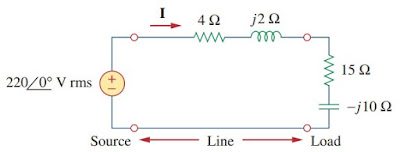Figure 2

Solution :
The total impedance is

The current through the circuit is

(a) For the source, the complex power is

From this, we obtain the real power as 2163.5 W and the reactive power as 910.8 VAR (leading).
(b) For the line, the voltage is

The complex power absorbed by the line is

or

That is, the real power is 455.4 W and the reactive power is 227.76 VAR (lagging).
(c) For the load, the voltage is

The complex power absorbed by the load is

The real power is 1708 W and the reactive power is 1139 VAR (leading). Note that Ss = Sline + SL, as expected. We have used the rms values of voltages and currents.

2. In the circuit of Figure.(3), Z1 = 60∠-30o Ω and Z2 = 40∠45o Ω. Calculate the total : (a) apparent power, (b) real power, (c) reactive power, and (d) pf, supplied by the source and seen by the source.Figure 3

Solution :
The current through Z1 is

while the current through Z2 is

The complex powers absorbed by the impedances are

The total complex power is

(a) The total apparent power is

(b) The total real power is

(c) The total reactive power is

(d) The pf = Pt/|St| = 462.4/481.6 = 0.96 (lagging).
We may cross check the result by finding the complex power Ss supplied by the source.

which is the same as before.

Have you understood what is conservation? Don’t forget to share and subscribe! Happy learning!

Reference:  Fundamentals of electric circuits by Charles K. Alexander and Matthew N. O. Sadiku.

Untuk Bahasa Indonesia baca Konservasi Daya di Rangkaian AC.